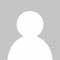Right clicking disabled.

# Calculate Surya Samvatsara

Here we shall discuss the Surya Samvatsara, which in Sanskrit lore is also termed as Mahakalpa and Maha Kalpa and which itself is the base (or root) , of the concept of Vedic Samvatsara …

This topic is in continuity with the previous one of “Sinusoidal paths” …

## 61-AA … What Surya Samvatsara … Mahakalpa or Maha Kalpa …

Surya Samvatsara is that solar time span which the sun takes “to complete 60 full-cycles which are esoterically stated to be resting within the mathematical symbol of infinity” …

Within some parts of Sanatan Dharma, time span of a Surya Samvatsara is also termed as “Mahakalpa (or Maha Kalpa) …

The end of Surya Samvatsara is told as Mahakalpa Pralaya (which can also be called as condition of Maha Kalpa Pralaya and the same can also be termed as the state of Surya Samvatsara Anta Pralaya) which means the great destruction which happens after the completion of three Brahma Kalpa)” …

The path of sun within the symbol of infinity is also the one which stands related to the 20 Brahma worlds (which in Sanskrit and other lore’s are also called as Brahmaloka) as the sun moves in its path whilst paying reverence to the divinities of each of these 20 Brahma worlds … We have already discussed this aspect in the previous topic of “Calculating Brahma Kalpa” …

Thus Surya Samvatsara is also equal to 120 revolutions of sun around the Milky way galaxy

### 61-AA-1 … Calculating Surya Samvatsara at middle time units …

This the time span is calculated as follows (and as per middle time units)…

One full-cycle of sun which runs within the symbolic path of infinity is of two solar revolution and thus it is of a time span as calculated below and as per the middle time units of precession of equinoxes

216 million human solar years x 2 = 432 million human solar years

Note: Value of 216 million human solar years is directly taken from the earlier of “Calculating solar revolution” … The time taken for one revolution of sun around the center of Milky way is also called as a galactic year

Thus basis above, 60 full cycles of solar path within the mathematical symbol of infinity and which determine a Surya Samvatsara shall be of a time span that is calculated below … Thus 60 full-cycles as are of a Surya Samvatsara shall be of a time span that is calculated below …

60 x 432 = 25,920 million human solar years = 25.92 billion human solar years

#### 61-AA-2 … Calculating Surya Samvatsara at Nadir time units …

This is calculated as follows …

{(Time span at middle time units / unitary value at middle time units) x unitary value at Nadir}

So basis above, is below calculation …

{(25,920 / 66.6666667) x 72} = 27,993.6 million human solar years = 27.9936  billion human solar years

Note: values of 66.6666667 and 72 are directly taken from calculations of an earlier topic of “unitary value of time” …

##### 61-AA-3 … Calculating Surya Samvatsara at Zenith time units of precession …

This is calculated as follows …

{(Time span at middle time units / unitary value at middle time units) x unitary value at Zenith}

So basis above, is below calculation …

{(25,920 / 66.6666667) x 61.3333333} = 23,846.4  million human solar years = 23.8464  billion human solar years

Note: values of 66.6666667 and 61.3333333 are taken from calculations of an earlier topic of “unitary value of time” …

###### 61-AA-4 … Calculating Surya Samvatsara at current time units of precession …

This is calculated as follows …

{(Time span at Middle time units / unitary value at Middle time units) x current unitary value}

So basis above, is below calculation …

{(25,920 / 66.6666667) x 71.6} = 27,838.08 million human solar years = 27.83808 billion human solar years

Note: values of 66.6666667 and 71.6 are directly taken from an earlier topic of “unitary value of time” …

Above time span of Surya Samvatsara is also used for determining the time which is remaining for the next “Nitya Pralaya (i.e. cyclic destruction of a major portion of this part of the universe)” and this is in addition to determining the “Astronomical age of universe” …

Surya Samvatsara is also the root knowledge of the Vedic Samvatsara Chakra (or in other words, the knowledge of Vedic Samvatsara) of 60 human solar years …

Time span of Surya Samvatsara is also the root of many other concepts including time spans which apply to “world of creator of allness (i.e. Time spans of Brahmaloka)” and of the Lifespan of any single creator (i.e. Brahma) …

Continues …

Share